A01_ProcessUnitsMeasures
 Data & Measurement
 Take every day before sleeping! Vocabulary Review Activities: (play them early and often!) Battleship JeopardyMillionaireJmblWrds BrainPop Animations and Practice Quizes Mean, Median, Mode, Range - Espanol Graphs - Espanol Coordinate Plane - Espanol Statistics- Espanol Measuring Matter - Espanol Precision and Accuracy- Espanol Metric Units- Espanol Vocabulary Science Skills Handbook 1999 -Graphing Appendix: Pages 202-214: What Is Science 1994 Measurement with theMetric System 1994: Chapter 2 Sections 1 & 2 Pages 38-61 Mathematics In Science Summary & Adapted Reading Worksheet Graphs In Science Summary & Adapted Reading Worksheet Scientists on the Cutting Edge Booklet Process Skills Packet
 Labs & Videos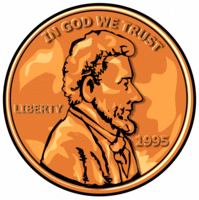Penny Observation Pre-Lab Worksheet Link PennyPrediction Pre-Lab Worksheet Link Hooda Math Graphing Gala Coordinate GameInteractiveSites.weebly Graphing Interactive Links BBC Bitesize Math Mean, Median, Mode, Range Frequency Diagrams Tables and Lists Graphs
 Data Packet Practice - Line, Bar, Circle Graphs VLC Graphs, Tables, Charts Video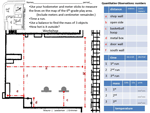Measure the Golden Center and other Quantitative Activities NCTM Illuminations SAS Curriculum Pathways Study Jams Animations and Practice Gizmos Nye Measurement Video Espanol Measurement Quiz Gizmos Grow a Plant- Experimental Write-Up IXL Math IXL Math
 Essential Vocabulary & Concepts
 Picture Core Knowledge or Concept Quantitative observations measure using numbers.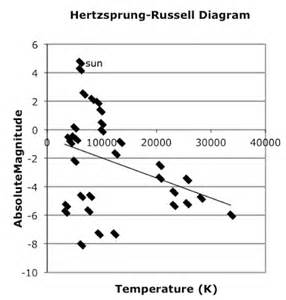Scatter Plot with Line of Best Fit Data Spread and Range measures how much variability is in a data setSpread is how much the data varies in valueRange = highest value - lowest value. i.e. for the set {35, 44, 73, 85} 85-35 = 50Range is often used to set up the scale of a graph using these values : maxima : the highest value minima : the lowest value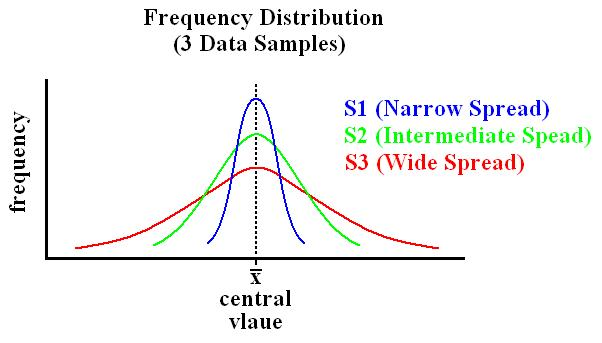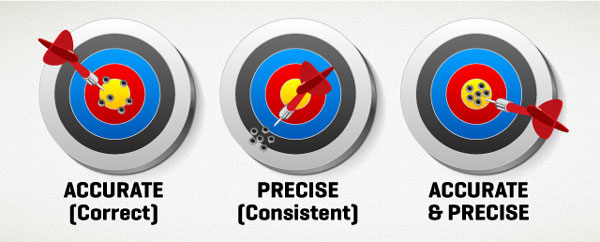Frequency Distribution - another way to look at spreadrelated to accuracy: How "correct" or close to target the values are.precision: how consistent the data is (close in value and predictable,) but not necessarily "correct."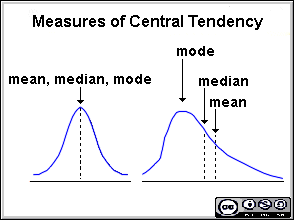Measures of Central TendencyMean - (most often used in data analysis) the sum of the values divided by the number of values. i.e. for the set {1,2,3,4,5} (1+2+3+4+5) / 5 = 15 / 5 = 3 is the averageMedian - the middle value in an ordered set i.e. in the set {1,2,3,4,5}, 3 is the middle valueMode - the most frequent value in a data set. i.e. in the set {1,1,1,2,2,3}, 1 occurs most often.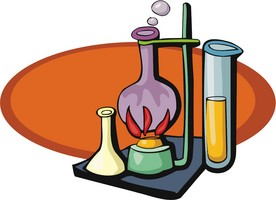Variables:  The three types are: Independent variable: the one thing you change to see if it has an effect on the dependent variable that you will measure Dependent variable: the one thing that is observed (measured) and recorded as data to check for change as the outcome Controlled variables: the things that stay the same so they don't have an effect on the outcome.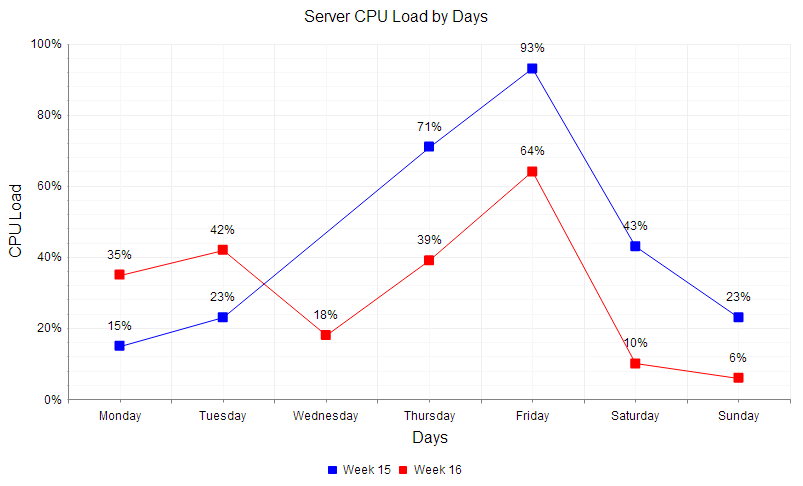Click on image Scatterplots and Line Graphs (2-dimensions) These coordinate plane graphs show pairs of data representing the Independent variable on the x-axis and the dependent variable on the y-axis Scatterplot: points (not connected) show correlation or cause and effect between independent and dependent variables.  May not have a 1-to-1 relationship in the data or other relationship such as change over time. May or may not show a trend that fits a line or curve. Line Graph: points are connected to show change in dependent variable as independent variable is changed.  Shows a 1-to-1 relationship in the data. Double Line Graph: shows multiple data sets (lines) on the same graph to compare data. Parts of a graph Title given as y versus x     i.e. Temperature over Time x-axis is always the independent variable y-axis is always the dependent variable labels on the axes show the name of the variable and the units of measurement i.e. distance in meters points - write the values on the graph for clarity and comparison, especially between lines origin : where the axes cross, does not have to be 0,0 but should be based on the range of your data and how you want to present it.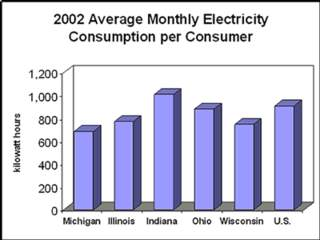Bar Graphs These coordinate plane graphs show categorized data as bars for comparison. May be horizontal or vertical. Categories Axis: on the axis at the base of the bar (usually the 'x' axis). i.e. month, color, type May not have a 1-to-1 relationship in the data. May not show relationship between categories Use color or patterns to show differences label each category and name the axis Values Axis: axis shows scale. Write number on the top of each bar for clarity and comparison. Often scaled with origin at 0 to show magnitude. label the axis with the name of the measure and scale Double Bar Graph: shows multiple data sets on the same graph to compare data. i.e. year-to-year, class-to-class, etc Histogram: shows frequency of value categories i.e. number of students at each shoe size Title given as Values  versus Categories     i.e. Students Favorite FlavorsPie Charts or Circle Graphs show categorized data as arc sections (slices from the center) for comparison to the whole. Scale: divide the circumference to appropriate part-to-whole divisions - fractions, percents, etc Categories: each section sized to the appropriate part-to-whole scale - fraction, percent, etc. Must be related to 100% or whole.. Label each category slice with the name and value Use colors or patterns to show differences Title given as Values  versus Categories     i.e. Students Favorite Flavors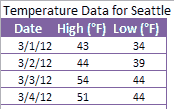Data Tables show data as numbers, often organized to show relationships (x,y pairs, etc.) often ordered by independent variable. headings: label columns and/or rows with name of data and units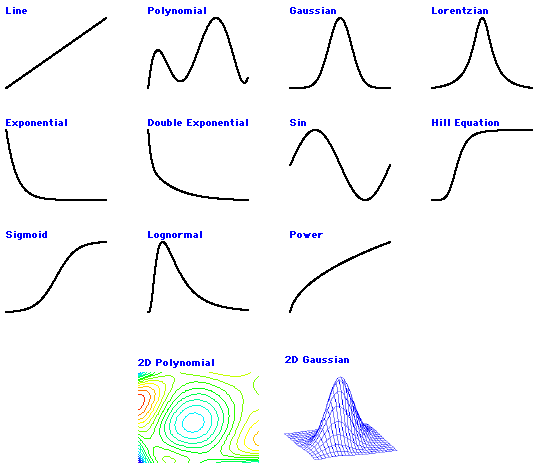Data Trends Linear non-linear increasing decreasing repeating cycle example names in picture to left Line of Best Fit - A mathematical process (usually with Excel) to find a line that fits the data. Used to find a correlation between independent and dependent values.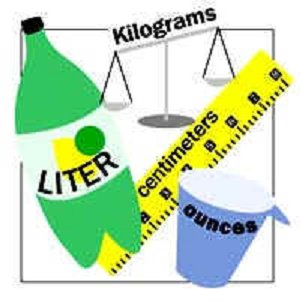Metric System (International System of Units, SI) a system of measurement based on the number 10.   A meter is the unit used to measure length, or the distance between two points.   A liter is the unit used to measure the volume of a liquid or the amount of space it takes up.    A gram is used to measure the amount of matter in an object.    Degrees Celsius measure temperature. Other GraphsBox and WhiskerPictographStem and Leaf